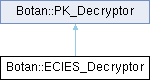Botan 3.0.0 Crypto and TLS for C&
Botan::ECIES_Decryptor Class Referencefinal

`#include <ecies.h>`

Inheritance diagram for Botan::ECIES_Decryptor:## Public Member Functions

secure_vector< uint8_t > decrypt (const uint8_t in[], size_t length) const

secure_vector< uint8_t > decrypt (std::span< const uint8_t > in) const

secure_vector< uint8_t > decrypt_or_random (const uint8_t in[], size_t length, size_t expected_pt_len, RandomNumberGenerator &rng) const

secure_vector< uint8_t > decrypt_or_random (const uint8_t in[], size_t length, size_t expected_pt_len, RandomNumberGenerator &rng, const uint8_t required_content_bytes[], const uint8_t required_content_offsets[], size_t required_contents) const

ECIES_Decryptor (const PK_Key_Agreement_Key &private_key, const ECIES_System_Params &ecies_params, RandomNumberGenerator &rng)

void set_initialization_vector (const InitializationVector &iv)
Set the initialization vector for the data encryption method.

void set_label (std::string_view label)
Set the label which is appended to the input for the message authentication code.

## Detailed Description

ECIES Decryption according to ISO 18033-2

Definition at line 276 of file ecies.h.

## ◆ ECIES_Decryptor()

 Botan::ECIES_Decryptor::ECIES_Decryptor ( const PK_Key_Agreement_Key & private_key, const ECIES_System_Params & ecies_params, RandomNumberGenerator & rng )
Parameters
 private_key the private key which is used for the key agreement ecies_params settings for ecies rng the random generator to use

Definition at line 343 of file ecies.cpp.

345 :
346 m_ka(key, ecies_params, false, rng),
347 m_params(ecies_params),
348 m_iv(),
349 m_label()
350 {
351 // ISO 18033: "If v > 1 and CheckMode = 0, then we must have gcd(u, v) = 1." (v = index, u= order)
352 if(!ecies_params.check_mode())
353 {
354 const BigInt& cofactor = m_params.domain().get_cofactor();
355 if(cofactor > 1 && gcd(cofactor, m_params.domain().get_order()) != 1)
356 {
357 throw Invalid_Argument("ECIES: gcd of cofactor and order must be 1 if check_mode is 0");
358 }
359 }
360
361 m_mac = m_params.create_mac();
362 m_cipher = m_params.create_cipher(Cipher_Dir::Decryption);
363 }
const EC_Group & domain() const
Definition: ecies.h:76
std::unique_ptr< Cipher_Mode > create_cipher(Cipher_Dir direction) const
creates an instance of the data encryption method
Definition: ecies.cpp:248
std::unique_ptr< MessageAuthenticationCode > create_mac() const
creates an instance of the message authentication code
Definition: ecies.cpp:243
const BigInt & get_cofactor() const
Definition: ec_group.cpp:560
const BigInt & get_order() const
Definition: ec_group.cpp:545
BigInt gcd(const BigInt &a, const BigInt &b)
Definition: numthry.cpp:220

## ◆ decrypt() [1/2]

 secure_vector< uint8_t > Botan::PK_Decryptor::decrypt ( const uint8_t in[], size_t length ) const
inherited

Decrypt a ciphertext, throwing an exception if the input seems to be invalid (eg due to an accidental or malicious error in the ciphertext).

Parameters
 in the ciphertext as a byte array length the length of the above byte array
Returns
decrypted message

Definition at line 20 of file pubkey.cpp.

21 {
23
24 secure_vector<uint8_t> decoded = do_decrypt(valid_mask, in, length);
25
27 throw Decoding_Error("Invalid public key ciphertext, cannot decrypt");
28
29 return decoded;
30 }

Referenced by Botan::KeyPair::encryption_consistency_check().

## ◆ decrypt() [2/2]

 secure_vector< uint8_t > Botan::PK_Decryptor::decrypt ( std::span< const uint8_t > in ) const
inlineinherited

Same as above, but taking a vector

Parameters
 in the ciphertext
Returns
decrypted message

Definition at line 102 of file pubkey.h.

103 {
104 return decrypt(in.data(), in.size());
105 }
secure_vector< uint8_t > decrypt(const uint8_t in[], size_t length) const
Definition: pubkey.cpp:20

## ◆ decrypt_or_random() [1/2]

 secure_vector< uint8_t > Botan::PK_Decryptor::decrypt_or_random ( const uint8_t in[], size_t length, size_t expected_pt_len, RandomNumberGenerator & rng ) const
inherited

Decrypt a ciphertext. If the ciphertext is invalid (eg due to invalid padding) or is not the expected length, instead returns a random string of the expected length. Use to avoid oracle attacks, especially against PKCS #1 v1.5 decryption.

Definition at line 81 of file pubkey.cpp.

85 {
86 return decrypt_or_random(in, length, expected_pt_len, rng,
87 nullptr, nullptr, 0);
88 }
secure_vector< uint8_t > decrypt_or_random(const uint8_t in[], size_t length, size_t expected_pt_len, RandomNumberGenerator &rng) const
Definition: pubkey.cpp:81

References Botan::PK_Decryptor::decrypt_or_random().

## ◆ decrypt_or_random() [2/2]

 secure_vector< uint8_t > Botan::PK_Decryptor::decrypt_or_random ( const uint8_t in[], size_t length, size_t expected_pt_len, RandomNumberGenerator & rng, const uint8_t required_content_bytes[], const uint8_t required_content_offsets[], size_t required_contents ) const
inherited

Decrypt a ciphertext. If the ciphertext is invalid (eg due to invalid padding) or is not the expected length, instead returns a random string of the expected length. Use to avoid oracle attacks, especially against PKCS #1 v1.5 decryption.

Additionally checks (also in const time) that: contents[required_content_offsets[i]] == required_content_bytes[i] for 0 <= i < required_contents

Used for example in TLS, which encodes the client version in the content bytes: if there is any timing variation the version check can be used as an oracle to recover the key.

Definition at line 33 of file pubkey.cpp.

40 {
41 const secure_vector<uint8_t> fake_pms = rng.random_vec(expected_pt_len);
42
43 uint8_t decrypt_valid = 0;
44 secure_vector<uint8_t> decoded = do_decrypt(decrypt_valid, in, length);
45
48
49 decoded.resize(expected_pt_len);
50
51 for(size_t i = 0; i != required_contents_length; ++i)
52 {
53 /*
54 These values are chosen by the application and for TLS are constants,
55 so this early failure via assert is fine since we know 0,1 < 48
56
57 If there is a protocol that has content checks on the key where
58 the expected offsets are controllable by the attacker this could
59 still leak.
60
61 Alternately could always reduce the offset modulo the length?
62 */
63
64 const uint8_t exp = required_content_bytes[i];
65 const uint8_t off = required_content_offsets[i];
66
67 BOTAN_ASSERT(off < expected_pt_len, "Offset in range of plaintext");
68
69 auto eq = CT::Mask<uint8_t>::is_equal(decoded[off], exp);
70
72 }
73
76
77 return decoded;
78 }
Definition: assert.h:54
static Mask< T > is_equal(T x, T y)
Definition: ct_utils.h:147
static Mask< T > is_zero(T x)
Definition: ct_utils.h:139

## ◆ set_initialization_vector()

 void Botan::ECIES_Decryptor::set_initialization_vector ( const InitializationVector & iv )
inline

Set the initialization vector for the data encryption method.

Definition at line 289 of file ecies.h.

290 {
291 m_iv = iv;
292 }

## ◆ set_label()

 void Botan::ECIES_Decryptor::set_label ( std::string_view label )
inline

Set the label which is appended to the input for the message authentication code.

Definition at line 295 of file ecies.h.

296 {
297 m_label = std::vector<uint8_t>(label.begin(), label.end());
298 }

The documentation for this class was generated from the following files: